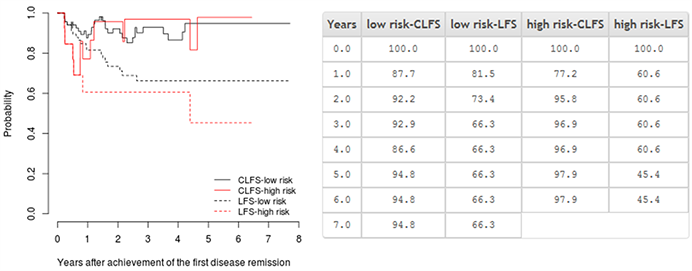# Examples of the current survival analysis using Czech data

The example of the current survival analysis is performed using data of 104 consecutive Czech chronic myeloid leukaemia (CML) patients in chronic phase treated by first-line imatinib between July 2003 and December 2011. All records were previously registered in the Czech database called INFINITY.

## Data set for unstratified analysis

Example data for the unstratified analysis has the following structure: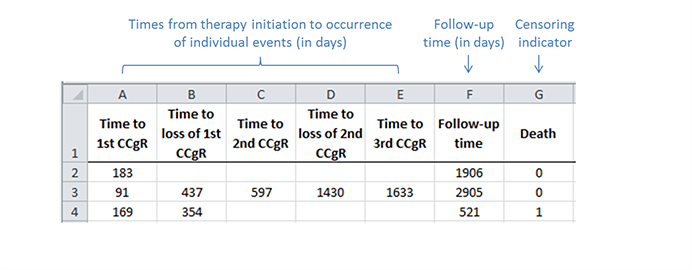Patients’ characteristics are summarized below. Out of the 104 patients, 88 achieved at least the first disease remission (i.e., the complete cytogenetic response (CCgR) in CML). The maximum number of remissions that were achieved during follow-up was 3. Eight patients died during the follow-up, which lasted 95 months at maximum.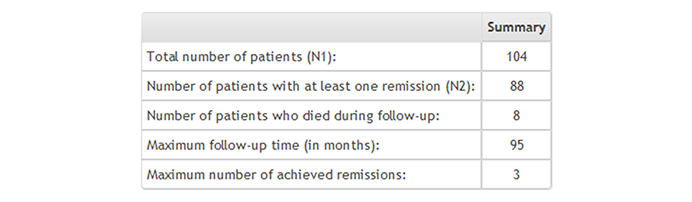The current cumulative incidence (CCI) and the common cumulative incidence (CI) point estimates are shown below. The estimated CCI and common CI at 5 years after starting imatinib therapy was 80.2% and 93.1%, respectively. Thus, the difference between the CCI and CI estimates reached 12.9%, which indicates that the common CI curve overestimates the probability of being alive and in remission after initiating the imatinib therapy.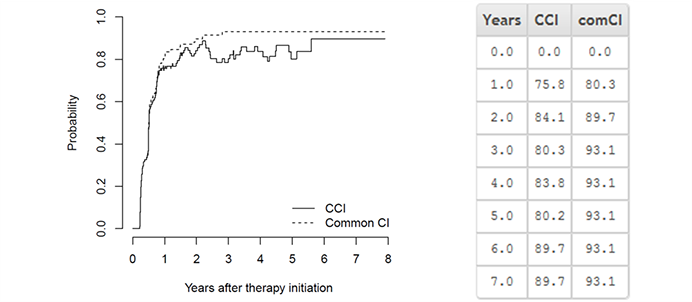The current leukaemia-free survival (CLFS) and the common leukaemia-free survival (LFS) point estimates are visualized below. The CLFS and LFS at 5 years after achieving the first CCgR were estimated as 95.3% and 61.8%, respectively. Therefore, the difference between the CLFS and LFS estimates reached 33.5%, which demonstrates that the LFS underestimates the probability of being alive and in remission after the achievement of first CCgR on the imatinib therapy.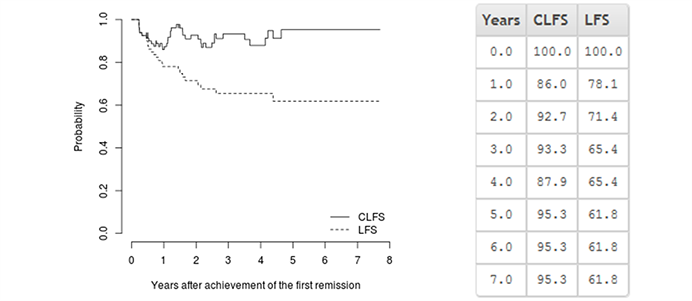## Data set for stratified analysis

Example data for the stratified analysis has the following structure: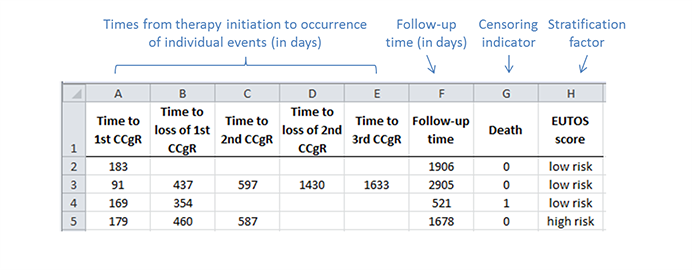Patients’ characteristics are summarized in the table below. Out of the 104 patients, 87 patients belonged to low-risk group according to the EUTOS score whereas 17 were classified as EUTOS high-risk patients. In total, 75 out of the 87 low-risk patients and 13 out of the 17 high-risk patients achieved at least the first disease remission. The maximum number of achieved remissions was 3 for both risk groups. During follow-up, which lasted 95 months or 83 months at maximum, 6 low-risk patients and 2 high-risk patients died, respectively.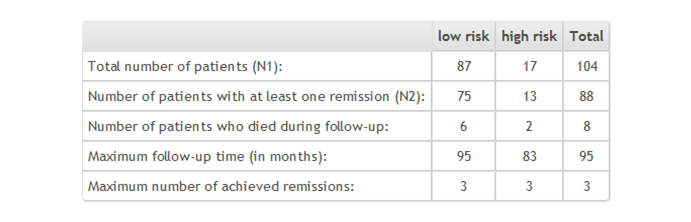The current cumulative incidence (CCI) point estimates according to the risk stratification are summarized below. The high-risk group had lower probability of being alive and in disease remission in time. For example, the estimated CCI at 5 years after starting imatinib therapy was 83.5% and 69.4% in the low-risk and high-risk group of patients, respectively.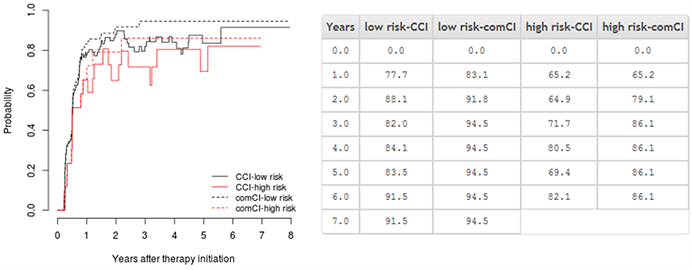The current leukaemia-free survival (CLFS) point estimates according to the risk stratification are shown below. For example, the estimated CLFS at 5 years after starting imatinib therapy was 94.8% and 97.9% in the low-risk and high-risk group of patients, respectively.